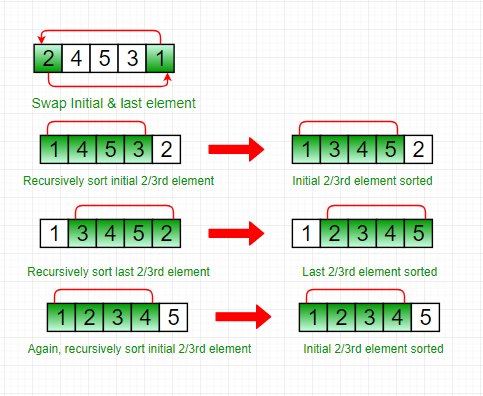# Stooge Sort

• Difficulty Level : Hard
• Last Updated : 14 Jun, 2022

Stooge Sort is a recursive sorting algorithm. It is not much efficient but interesting sorting algorithm. It generally divides the array into two overlapping parts (2/3 each). After that it performs sorting in first 2/3 part and then it performs sorting in last 2/3 part. And then, sorting is done on first 2/3 part to ensure that the array is sorted.

The key idea is that sorting the overlapping part twice exchanges the elements between the other two sections accordingly.

Approach:

Step 1: If value at index 0 is greater than value at last index, swap them.
Step 2: Recursively,

• Stooge sort the initial 2/3rd of the array.
• Stooge sort the last 2/3rd of the array.
• Stooge sort the initial 2/3rd again to confirm.

NOTE: Always take the ceil of ((2/3)*N) for selecting elements.

Illustration:

Lets consider an example: arr[] = {2, 4, 5, 3, 1}

• Step1: Initially, First and last elements are compared and if last is greater than first then they are swapped.
• Step2: Now, recursively sort initial 2/3rd of the elements as shown below:
• Step3: Then, recursively sort last 2/3rd of the elements, as shown below:
• Step4: Again, sort the initial 2/3rd of the elements to confirm final data is sorted.
• Resulted array:Below is the implementation for the above approach:

## C++

 `// C++ code to implement stooge sort``#include ``using` `namespace` `std;`` ` `// Function to implement stooge sort``void` `stoogesort(``int` `arr[], ``int` `l, ``int` `h)``{``    ``if` `(l >= h)``        ``return``;`` ` `    ``// If first element is smaller than last,``    ``// swap them``    ``if` `(arr[l] > arr[h])``        ``swap(arr[l], arr[h]);`` ` `    ``// If there are more than 2 elements in``    ``// the array``    ``if` `(h - l + 1 > 2) {``        ``int` `t = (h - l + 1) / 3;`` ` `        ``// Recursively sort first 2/3 elements``        ``stoogesort(arr, l, h - t);`` ` `        ``// Recursively sort last 2/3 elements``        ``stoogesort(arr, l + t, h);`` ` `        ``// Recursively sort first 2/3 elements``        ``// again to confirm``        ``stoogesort(arr, l, h - t);``    ``}``}`` ` `// Driver Code``int` `main()``{``    ``int` `arr[] = { 2, 4, 5, 3, 1 };``    ``int` `n = ``sizeof``(arr) / ``sizeof``(arr);`` ` `    ``// Calling Stooge Sort function to sort``    ``// the array``    ``stoogesort(arr, 0, n - 1);`` ` `    ``// Display the sorted array``    ``for` `(``int` `i = 0; i < n; i++)``        ``cout << arr[i] << ``" "``;`` ` `    ``return` `0;``}`

## Java

 `// Java program to implement stooge sort``import` `java.io.*;`` ` `public` `class` `stooge {``    ``// Function to implement stooge sort``    ``static` `void` `stoogesort(``int` `arr[], ``int` `l, ``int` `h)``    ``{``        ``if` `(l >= h)``            ``return``;`` ` `        ``// If first element is smaller``        ``// than last, swap them``        ``if` `(arr[l] > arr[h]) {``            ``int` `t = arr[l];``            ``arr[l] = arr[h];``            ``arr[h] = t;``        ``}`` ` `        ``// If there are more than 2 elements in``        ``// the array``        ``if` `(h - l + ``1` `> ``2``) {``            ``int` `t = (h - l + ``1``) / ``3``;`` ` `            ``// Recursively sort first 2/3 elements``            ``stoogesort(arr, l, h - t);`` ` `            ``// Recursively sort last 2/3 elements``            ``stoogesort(arr, l + t, h);`` ` `            ``// Recursively sort first 2/3 elements``            ``// again to confirm``            ``stoogesort(arr, l, h - t);``        ``}``    ``}`` ` `    ``// Driver Code``    ``public` `static` `void` `main(String args[])``    ``{``        ``int` `arr[] = { ``2``, ``4``, ``5``, ``3``, ``1` `};``        ``int` `n = arr.length;`` ` `        ``stoogesort(arr, ``0``, n - ``1``);`` ` `        ``for` `(``int` `i = ``0``; i < n; i++)``            ``System.out.print(arr[i] + ``" "``);``    ``}``}``// Code Contributed by Mohit Gupta_OMG <(0_o)>`

## Python3

 `# Python program to implement stooge sort`` ` `def` `stoogesort(arr, l, h):``    ``if` `l >``=` `h:``        ``return``  ` `    ``# If first element is smaller``    ``# than last, swap them``    ``if` `arr[l]>arr[h]:``        ``t ``=` `arr[l]``        ``arr[l] ``=` `arr[h]``        ``arr[h] ``=` `t``  ` `    ``# If there are more than 2 elements in``    ``# the array``    ``if` `h``-``l ``+` `1` `> ``2``:``        ``t ``=` `(``int``)((h``-``l ``+` `1``)``/``3``)``  ` `        ``# Recursively sort first 2 / 3 elements``        ``stoogesort(arr, l, (h``-``t))``  ` `        ``# Recursively sort last 2 / 3 elements``        ``stoogesort(arr, l ``+` `t, (h))``  ` `        ``# Recursively sort first 2 / 3 elements``        ``# again to confirm``        ``stoogesort(arr, l, (h``-``t))``  ` ` ` `# deriver ``arr ``=` `[``2``, ``4``, ``5``, ``3``, ``1``]``n ``=` `len``(arr)`` ` `stoogesort(arr, ``0``, n``-``1``)``  ` `for` `i ``in` `range``(``0``, n):``    ``print``(arr[i], end ``=` `' '``)`` ` `# Code Contributed by Mohit Gupta_OMG <(0_o)>`

## C#

 `// C# program to implement stooge sort``using` `System;`` ` `class` `GFG {``     ` `    ``// Function to implement stooge sort``    ``static` `void` `stoogesort(``int``[] arr,``                            ``int` `l, ``int` `h)``    ``{``        ``if` `(l >= h)``            ``return``;`` ` `        ``// If first element is smaller``        ``// than last, swap them``        ``if` `(arr[l] > arr[h]) {``            ``int` `t = arr[l];``            ``arr[l] = arr[h];``            ``arr[h] = t;``        ``}`` ` `        ``// If there are more than 2 ``        ``// elements in the array``        ``if` `(h - l + 1 > 2) {``            ``int` `t = (h - l + 1) / 3;`` ` `            ``// Recursively sort first ``            ``// 2/3 elements``            ``stoogesort(arr, l, h - t);`` ` `            ``// Recursively sort last``            ``// 2/3 elements``            ``stoogesort(arr, l + t, h);`` ` `            ``// Recursively sort first ``            ``// 2/3 elements again to ``            ``// confirm``            ``stoogesort(arr, l, h - t);``        ``}``    ``}`` ` `    ``// Driver Code``    ``public` `static` `void` `Main()``    ``{``        ``int``[] arr = { 2, 4, 5, 3, 1 };``        ``int` `n = arr.Length;`` ` `        ``// Calling Stooge Sort function``        ``// to sort the array``        ``stoogesort(arr, 0, n - 1);`` ` `        ``// Display the sorted array``        ``for` `(``int` `i = 0; i < n; i++)``            ``Console.Write(arr[i] + ``" "``);``    ``}``}`` ` `// This code is contributed by Sam007.`

## Javascript

 ``

Output

`1 2 3 4 5 `

The running time complexity of stooge sort can be written as,
T(n) = 3T(3n/2) + ?(1)

Solution of above recurrence is O(n(log3/log1.5)) = O(n2.709), hence it is slower than even bubble sort(n^2).

### Check out DSA Self Paced Course

This article is contributed by DANISH KALEEM. If you like GeeksforGeeks and would like to contribute, you can also write an article using write.geeksforgeeks.org or mail your article to review-team@geeksforgeeks.org. See your article appearing on the GeeksforGeeks main page and help other Geeks.

My Personal Notes arrow_drop_up